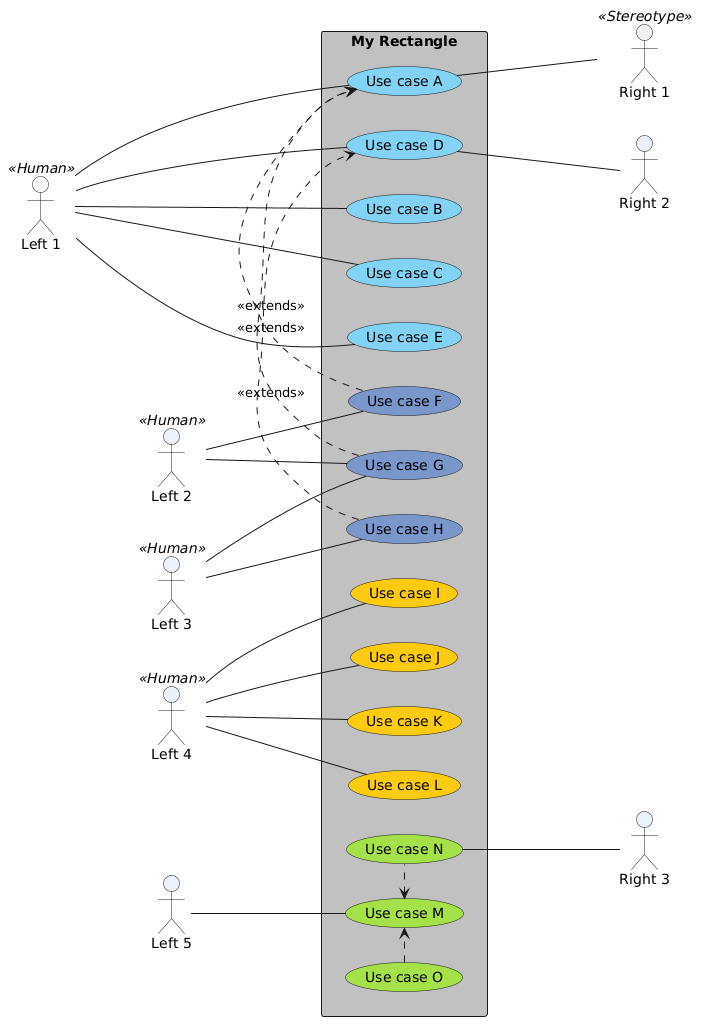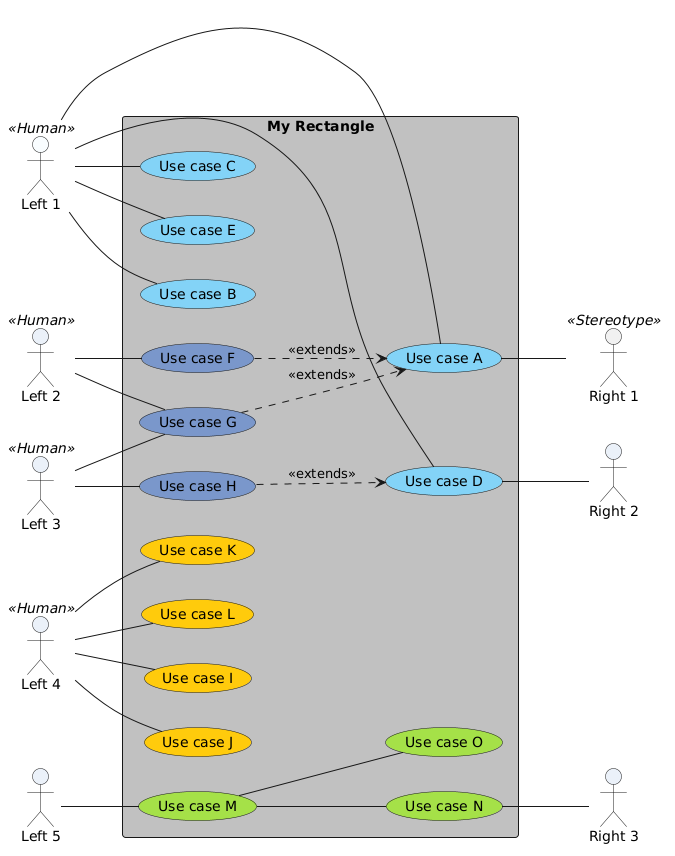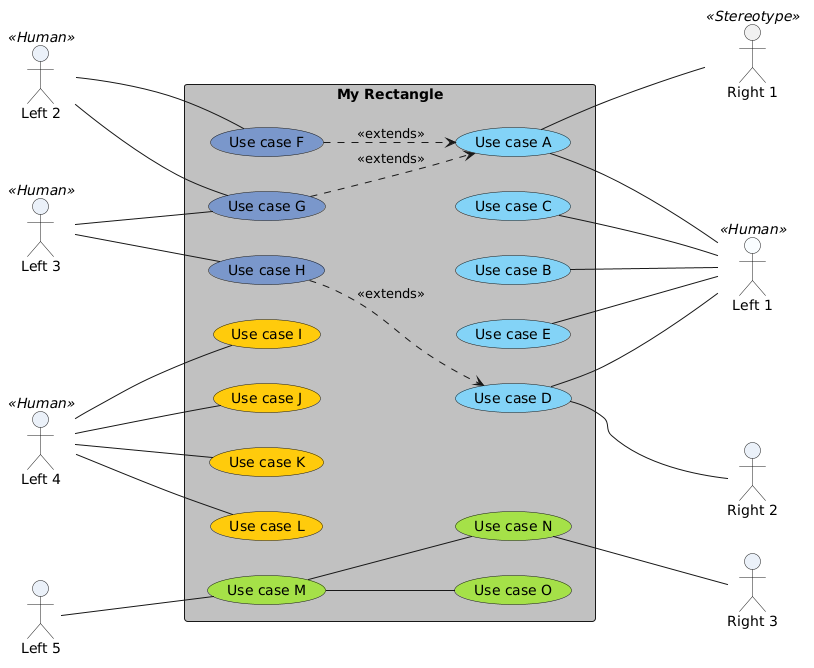# How to make Actors appear on the left AND Right of a Use Case diagram?

edited Feb 14, 2019

In the following example code, the Actors "Right 1" and "Right 2" will appear on the Left. How do I make them appear on the right, and adjacent to (horizontal to) their Use cases?

PlantUML does not seem to allow it. Only "Right 3" is positioned on the right.

```@startuml

' ======= the actors =========

actor :Left 1: as Left1 << Human >> #f8fdff
actor :Right 1: as Right1 << Stereotype >>
actor :Left 2: as Left2 << Human >> #eaf0f9

actor :Left 3: as Left3 << Human >> #eaf0f9
actor :Left 4: as Left4 << Human >> #eaf0f9

actor :Left 5: as Left5  #eaf0f9
actor :Right 3: as Right3  #eaf0f9
actor :Right 2: as Right2  #eaf0f9

rectangle "My Rectangle" #C0C0C0 {
left to right direction

' ====== the use cases =========

(Use case A) as (UseCaseA) #83d3f6
(Use case B) as (UseCaseB) #83d3f6
(Use case C) as (UseCaseC) #83d3f6
(Use case D) as (UseCaseD) #83d3f6
(Use case E) as (UseCaseE) #83d3f6

(Use case F) as (UseCaseF) #7a97ca
(Use case G) as (UseCaseG) #7a97ca
(Use case H) as (UseCaseH) #7a97ca

(Use case I) as (UseCaseI) #ffcb0c
(Use case J) as (UseCaseJ) #ffcb0c
(Use case K) as (UseCaseK) #ffcb0c
(Use case L) as (UseCaseL) #ffcb0c

(Use case M) as (UseCaseM) #a4e148
(Use case N) as (UseCaseN) #a4e148
(Use case O) as (UseCaseO) #a4e148

' ====== the use case links =========

Left1 -- (UseCaseA)
Left1 -- (UseCaseB)
Left1 -- (UseCaseC)
Left1 -- (UseCaseD)
Left1 -- (UseCaseE)

Left2 -- (UseCaseF)
Left2 -- (UseCaseG)
(UseCaseF) .> (UseCaseA) : << extends >>
(UseCaseG) .> (UseCaseA) : << extends >>

Left3 -- (UseCaseG)
Left3 -- (UseCaseH)
(UseCaseH) .> (UseCaseD) : << extends >>

Left4 -- (UseCaseI)
Left4 -- (UseCaseJ)
Left4 -- (UseCaseK)
Left4 -- (UseCaseL)

Left5 -- (UseCaseM)
(UseCaseM) -- (UseCaseN)
(UseCaseM) -- (UseCaseO)
Right3 -up- (UseCaseN)

'TODO: These last 2 should be positioned on the right hand side. How to do it?
Right1 -- (UseCaseA)
Right2 -- (UseCaseD)

'These do not work. PlantUML will put the Actors far away at the bottom
'(UseCaseA) -- Right1
'(UseCaseD) -- Right2
}

@enduml

```

Preview of diagram:

answered Feb 13, 2019 by (260 points)
edited Feb 28, 2019 by ibey7804

I have figured out a workaround. It's based on the following things:

• Use "together { }", to force the last 3 Use-Cases to be grouped togther
• For the last 3 Use-Cases, convert the hard lines to short dotted lines, using ".>" to create the links. It seems that the normal plain lines will ruin the positioning.

This should now work to positon some Actors on the right, as in the example below:

```@startuml

' ======= the actors =========

actor :Left 1: as Left1 << Human >> #f8fdff
actor :Right 1: as Right1 << Stereotype >>
actor :Left 2: as Left2 << Human >> #eaf0f9

actor :Left 3: as Left3 << Human >> #eaf0f9
actor :Left 4: as Left4 << Human >> #eaf0f9

actor :Left 5: as Left5  #eaf0f9
actor :Right 3: as Right3  #eaf0f9
actor :Right 2: as Right2  #eaf0f9

rectangle "My Rectangle" #C0C0C0 {
left to right direction

' ====== the use cases =========

(Use case A) as (UseCaseA) #83d3f6
(Use case B) as (UseCaseB) #83d3f6
(Use case C) as (UseCaseC) #83d3f6
(Use case D) as (UseCaseD) #83d3f6
(Use case E) as (UseCaseE) #83d3f6

(Use case F) as (UseCaseF) #7a97ca
(Use case G) as (UseCaseG) #7a97ca
(Use case H) as (UseCaseH) #7a97ca

(Use case I) as (UseCaseI) #ffcb0c
(Use case J) as (UseCaseJ) #ffcb0c
(Use case K) as (UseCaseK) #ffcb0c
(Use case L) as (UseCaseL) #ffcb0c

' Prevent PlantUML from re-ordering these items
together {
(Use case M) as (UseCaseM) #a4e148
(Use case N) as (UseCaseN) #a4e148
(Use case O) as (UseCaseO) #a4e148
}

' ==== the use case links. Note '---' means longer line ======

' These Actors are positioned on the left hand side.
' Note the Actor is referenced first, and the Use-Case second.
Left1 --- (UseCaseA)
Left1 -- (UseCaseB)
Left1 -- (UseCaseC)
Left1 -- (UseCaseD)
Left1 -- (UseCaseE)

Left2 -- (UseCaseF)
Left2 -- (UseCaseG)
(UseCaseF) .> (UseCaseA) : << extends >>
(UseCaseG) .> (UseCaseA) : << extends >>

Left3 -- (UseCaseG)
Left3 -- (UseCaseH)
(UseCaseH) .> (UseCaseD) : << extends >>

Left4 -- (UseCaseI)
Left4 -- (UseCaseJ)
Left4 -- (UseCaseK)
Left4 -- (UseCaseL)

Left5 -- (UseCaseM)

' These dotted lines will cause a better placement of the
' Use-Cases, compared to using the normal non-dotted lines.
(UseCaseM) <. (UseCaseN)
(UseCaseO) .> (UseCaseM)

' These Actors are positioned on the right hand side.
' Note the Use-Case is referenced first, and the Actor second.
(UseCaseN) --- Right3
(UseCaseA) --- Right1
(UseCaseD) --- Right2
}

@enduml

```answered Feb 28, 2019 by (300 points)

This is the best I manage to get :answered Feb 28, 2019 by (260 points)

Here is another way, with the following changes:

• Use long lines. Most of them are "---"
• Position Left1 on the Right
• Use "together" for 2 groups of Use-Cases at the top
```@startuml
' ======= the actors =========

actor :Left 1: as Left1 << Human >> #f8fdff
actor :Right 1: as Right1 << Stereotype >>
actor :Left 2: as Left2 << Human >> #eaf0f9

actor :Left 3: as Left3 << Human >> #eaf0f9
actor :Left 4: as Left4 << Human >> #eaf0f9

actor :Left 5: as Left5  #eaf0f9
actor :Right 3: as Right3  #eaf0f9
actor :Right 2: as Right2  #eaf0f9

rectangle "My Rectangle" #C0C0C0 {
left to right direction

' ====== the use cases =========

'Don't let PlantUML re-order these items. So use "together"
together {
(Use case A) as (UseCaseA) #83d3f6
(Use case B) as (UseCaseB) #83d3f6
(Use case C) as (UseCaseC) #83d3f6
(Use case D) as (UseCaseD) #83d3f6
(Use case E) as (UseCaseE) #83d3f6
}

together {
(Use case F) as (UseCaseF) #7a97ca
(Use case G) as (UseCaseG) #7a97ca
(Use case H) as (UseCaseH) #7a97ca
}

(Use case I) as (UseCaseI) #ffcb0c
(Use case J) as (UseCaseJ) #ffcb0c
(Use case K) as (UseCaseK) #ffcb0c
(Use case L) as (UseCaseL) #ffcb0c

(Use case M) as (UseCaseM) #a4e148
(Use case N) as (UseCaseN) #a4e148
(Use case O) as (UseCaseO) #a4e148

' ====== the use case links (use long lines) =========

' Left1 Actor is now positioned on the Right, for better layout
(UseCaseA) --- Left1
(UseCaseB) --- Left1
(UseCaseC) --- Left1
(UseCaseD) --- Left1
(UseCaseE) --- Left1

Left2 --- (UseCaseF)
Left2 --- (UseCaseG)
(UseCaseF) ..> (UseCaseA) : << extends >>
(UseCaseG) .> (UseCaseA) : << extends >>

Left3 --- (UseCaseG)
Left3 --- (UseCaseH)
(UseCaseH) .> (UseCaseD) : << extends >>

Left4 --- (UseCaseI)
Left4 --- (UseCaseJ)
Left4 --- (UseCaseK)
Left4 --- (UseCaseL)

Left5 --- (UseCaseM)
(UseCaseM) -- (UseCaseN)
(UseCaseM) -- (UseCaseO)
Right3 -up-- (UseCaseN)

(UseCaseA) --- Right1
(UseCaseD) --- Right2
}
@enduml```

Preview of diagram:

http://www.plantuml.com/plantuml/uml/XPNTRjf048Nl_HGZV94YbGWmD0IbAE6d47wWIfG7sDXZOjLuaRrMYwgyUzT3Z3sKTPOR7vzlpixAkyjzeaIiqbreNS7tum3rXY1S9UE4Nj6mB7e3_INw2bfz40baZosukuDPkXCH30PW-ptVy_sSVGgsRpbCpqG_AunHgiCUTGcBRPzYspmMXT_qRtaFpWbsBi6CxPpOpkV1tHFSXPpWAtAA5Ja6f5qWxGAnOdILYBOXGcrrWATSrS0UDRCV_BL0Zp3hGqc8ASiBih90HXQPLr3wJcc2u8e4sQSYQYoZAwMJ5FmCHQHULak8iI5Z3-EiKjS52dV9DJnBIgafkKLjn3MgpzMn8nfVNZucWuSwY8JqI4kjx9xZEVwt2-YGeSCgTCJGKHKwPkYu2fqmTCBHT-lpnVvW0LhPD-BsnXKNq2b3frNeZA4pXb9RZ7vaj5QsxxklJVSCcpDiRi8M35kOi2N3bWLsngqOfvKjEjZgzCwmDSFM9cp3i4s1cJS_X47qIzTaEfJHDjEOrFyx4XytrWCTr221IFw6lKo2x6oX1pAYL3gqNy7Np2igXJ64uY1JHHcbFTzeD8w1p1YQZ97969kCITaWXwv6UbbiHuCn9QF4mVNreDn-Fxi9yO_2o4jWC2XeAePAkEZ6EPlKOCp8A2JB7rVaTrZCOzrWp4t6mcGiwyKaNUQigAGaeMIkgymDcVaVGoFTyy9B6uZOY_k1dBRrRjrZvAMxy1y0